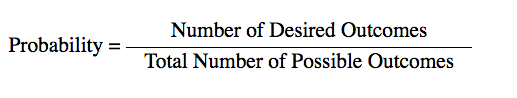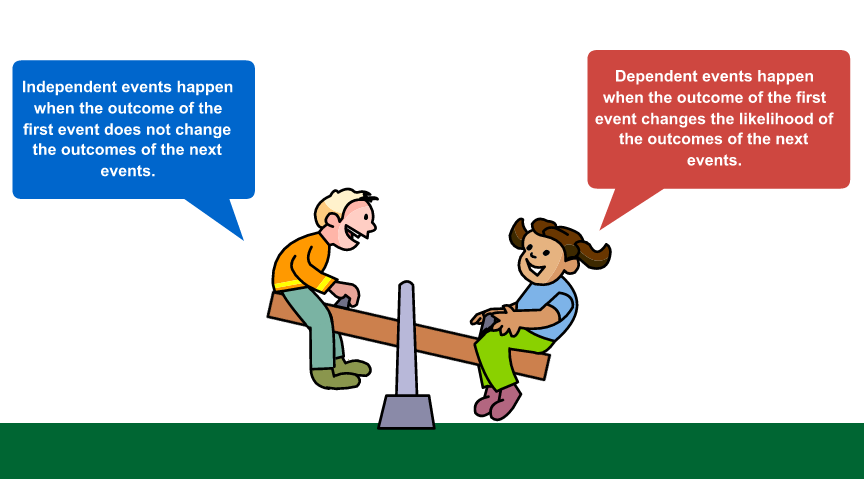# Introduction

In previous resources, you've used probability to describe the likelihood of events occurring. You’ve also used probability to calculate the likelihood of two consecutive events occurring.

Probability is formally defined as the ratio of the number of desired outcomes (what you want to happen) to the number of total possible outcomes (what could possibly happen). Probability is a ratio, so it can be expressed as a fraction, a decimal, or a percent.We can represent the probability of an event using the notation below.

P(event)For example, out of a dozen cookies, there are 9 chocolate chip cookies and 3 sugar cookies. The probability of randomly choosing a chocolate chip cookie from the cookie jar is $\frac{9}{12}$. Since this is only one event occurring, this is called a simple event.

Using probability notation, this would be written as follows.

P(chocolate chip) = $\frac{9}{12}$

Probabilities are ratios, so they can be expressed as fractions, decimals, or percents.

P(chocolate chip) = $\frac{9}{12}$

P(chocolate chip) = 0.75

P(chocolate chip) = 75%

Suppose that someone selects more than one cookie from the cookie jar without looking. What is the probability that these cookies will all be chocolate chip cookies? In this resource, you will investigate different ways to answer that question.

# Distinguishing Between Independent and Dependent ProbabilityThe relationship between multiple events that occur is important. The key question that you need to think about is, “does the first event influence the outcome of the next event?”

For example, consider a pair of number cubes. One cube is red and the other cube is white. Let’s assume that both number cubes are fair cubes; that is, each number on each cube has an equal chance of landing on the top face.

If you roll both cubes, does the number that appears on the top face of the red number cube affect the number that appears on the top face of the white number cube? Why do you think that is the case?

Sort the following situations into two categories: situations where the outcome of the second event depends on the outcome of the first event, and situations where the outcome of the second event does not depend on the outcome of the first event.

When you have a series of compound events where the outcome of the first event affects the outcomes of the subsequent events, these events are called dependent events. We can say that the outcome of the second event depends on the outcome of the first event.

When you have a series of compound events where the outcome of the first event does not affect the outcomes of the subsequent events, these events are called independent events. We can say that the outcome of the second event is independent of the outcome of the first event.

Practice

Determine whether the following events are independent events or dependent events.

1. Tina flips a coin and rolls a number cube.
2. Herb reaches into a box containing 12 instant oatmeal packages and randomly selects one package of cinnamon oatmeal and one package of maple oatmeal.
3. Shirley reaches into a box containing 12 instant oatmeal packages and randomly selects one package of blueberry oatmeal. She replaces the package, and then randomly selects another package of blueberry oatmeal.
4. Mrs. Siddiqi is an inspector on a computer assembly line. Of the 20 computers on the assembly line, she randomly selects 3 computers for detailed inspection.

# Determining the Probability of Dependent Events

In the previous section, you investigated the differences between independent events and dependent events.Suppose a bag contains several color tiles: 4 red tiles, 3 green tiles, 6 yellow tiles, and 2 blue tiles.Cynthia reaches into the bag without looking and randomly pulls out one color tile. Use the bag containing the tiles to determine the probability of each color being drawn.

The probabilities that you just identified are for the simple event of one color tile being drawn. Suppose, however, that Cynthia draws two tiles at the same time. Because two simple events will occur, these events become compound events.

Pause and Reflect

1. When Cynthia draws the first tile, will that affect the possibilities of the tile that she can draw for the second tile? Why or why not?
2. Are these events independent events or dependent events? How do you know?

To determine the probability of a set of compound events, you must first identify the probabilities of each of the events occurring by themselves.

Remember the definition of probability.Let's go back to the bag of tiles. Cynthia will draw two tiles from the bag at the same time.

Drag the fraction that represents the probability of each of the following events to the space indicated. For example, for the first line, drag the fraction representing the probability of Cynthia drawing a red tile first to the box in the Probability of First Event column. Drag the fraction representing the probability of Cynthia drawing a green tile second to the box in the Probability of Second Event column.

Now, calculate the probability of both events occurring for each player by multiplying the probability of the first event and second event together.

Practice

A word game consists of tiles where each tile has one letter. There are 42 vowels and 26 consonants. The 42 vowel tiles are distributed as shown in the table.

 Letter Tiles Letter A E I O U Number of Tiles 9 12 9 8 4

Samuel draws 2 tiles from the bag containing all of the letter tiles.

# Summary

In this resource, you distinguished between independent and dependent events with respect to determining the probability of those events.

Independent events are two or more events that occur in sequence where the outcome of the first event does not affect the outcome of the events that follow. You can calculate the probability of a series of independent events by using the Multiplication Rule of Probability:

P(A and B) = P(A) x P(B)

Dependent events are two or more events that occur in sequence where the outcome of the first event does affect the outcome of the events that follow. You can calculate the probability of a series of dependent events by using the Multiplication Rule of Probability:

P(A and B) = P(A) x P(B|A)

With this notation for dependent events, P(B|A) means “the probability that event B will happen given that event A already happened.” This distinction is important, because with dependent probability, the outcomes for event B are dependent upon event A. In other words, the outcome of event A influences the possible outcomes for event B.